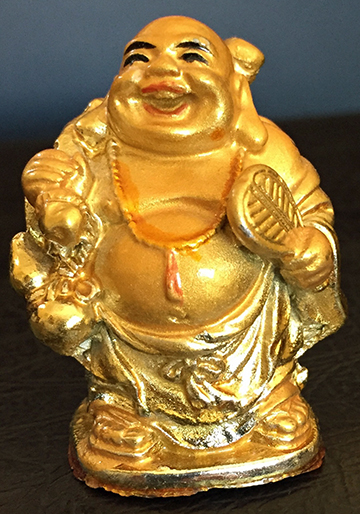# A Generous Uncle?When my daughter was 6 years old, her uncle gave her this figurine, a couple of inches tall.

She asked if it was made of pure gold. Well, let’s see how much it would cost if it were.
At the time of this writing, gold costs about \$37.88 per gram. The density of gold is 19.3 g/cm3. So now we just need to figure out the volume of the figurine in cubic centimeters. For this, we use a measuring cup:

So the volume of the figurine is about 25ml which is the same as 25 cm3. This is all much more fun without a calculator, so, to keep things simple, let’s approximate the density of gold to 20 g/cm3, and the price of gold to \$40/gram. So the figurine weights about 25 cm3 * 20 g/ cm3 = 500 grams (yes, that’s about a pound for a figurine only a few inches tall, since gold is very dense; it’s more than twice as dense as iron). The cost of the figurine, and that’s not counting the work to make it and any mark up by the seller, would then be 500 grams* \$40/gram= \$20,000. Giving his life’s savings to a 6 year old? Maybe not.